# ISEE Lower Level Quantitative : How to find the area of a parallelogram

## Example Questions

### Example Question #51 : Plane Geometry

What area of the rectangle is filled in?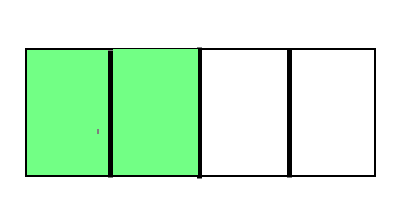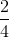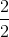Explanation:

Area is the amount of space within the perimeter of a two-dimensional shape. The rectangle is split intopieces andof those pieces are filled in.of the area of this rectangle is filled in.

### Example Question #5 : Find Area By Partitioning Shapes Into Equal Parts: Ccss.Math.Content.3.G.A.2

What area of the rectangle is filled in?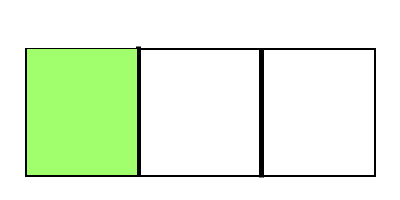Explanation:

Area is the amount of space within the perimeter of a two-dimensional shape. The rectangle is split intopieces andof those pieces is filled in.of the area of this rectangle is filled in.

### Example Question #31 : Geometry

What area of the rectangle is filled in?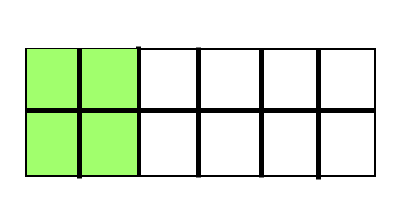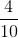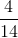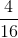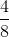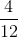Explanation:

Area is the amount of space within the perimeter of a two-dimensional shape. The rectangle is split intopieces andof those pieces are filled in.of the area of this rectangle is filled in.

### Example Question #3 : Find Area By Partitioning Shapes Into Equal Parts: Ccss.Math.Content.3.G.A.2

What area of the rectangle is filled in?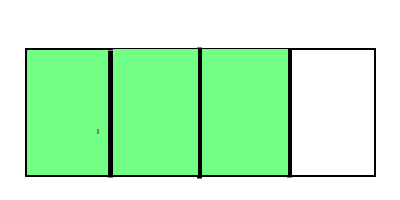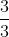Explanation:

Area is the amount of space within the perimeter of a two-dimensional shape. The rectangle is split intopieces andof those pieces are filled in.of the area of this rectangle is filled in.

### Example Question #33 : Geometry

What area of the rectangle is filled in?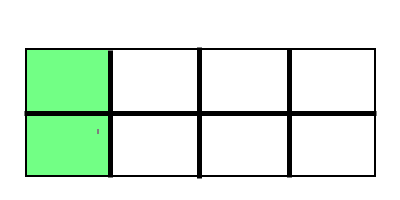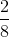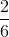Explanation:

Area is the amount of space within the perimeter of a two-dimensional shape. The rectangle is split intopieces andof those pieces are filled in.of the area of this rectangle is filled in.

### Example Question #31 : Geometry

What area of the rectangle is filled in?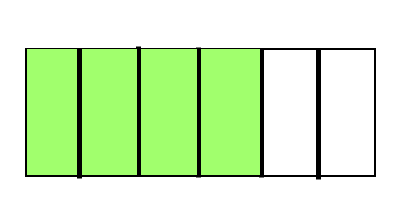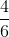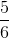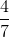Explanation:

Area is the amount of space within the perimeter of a two-dimensional shape. The rectangle is split intopieces andof those pieces are filled in.of the area of this rectangle is filled in.

### Example Question #52 : Plane Geometry

What area of the rectangle is filled in?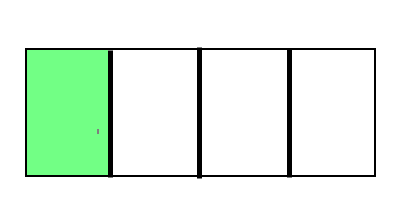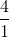Explanation:

Area is the amount of space within the perimeter of a two-dimensional shape. The rectangle is split intopieces andof those pieces is filled in.of the area of this rectangle is filled in.

### Example Question #51 : Plane Geometry

How many square units make up the area of the shape below?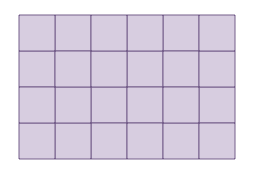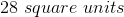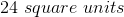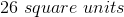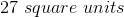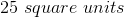Explanation:

The shape is made up of unit squares. We can count the number of squares within the shape to find the area.

There aresquares within the shape.

### Example Question #1 : Unit Squares Used To Measure Area: Ccss.Math.Content.3.Md.C.5a

How many square units make up the area of the shape below?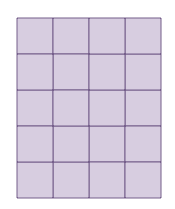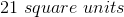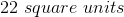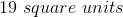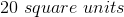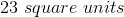Explanation:

The shape is made up of unit squares. We can count the number of squares within the shape to find the area.

There aresquares within the shape.

### Example Question #61 : Plane Geometry

How many square units make up the area of the shape below?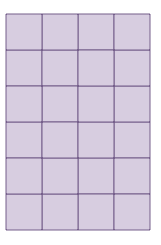There aresquares within the shape.